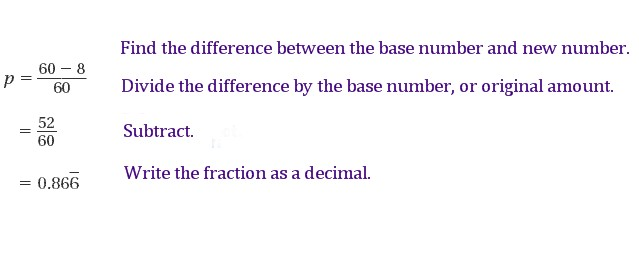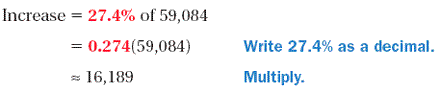Percent Change

Percent Change

The "percent change," or "percent of change" shows how much a quantity has increased or decreased in relation to the original amount. When the new amount is greater than the original amount, the percent change is called a percent of increase, or just "percent increase." When the new amount is less than the original amount, it is called a percent of decrease, or "percent decrease."

Percent Change

Use the following equation to find the percent change:Percent Decrease

Example

During the summer, a bear's heart rate is about 60 beats per minute, but it can drop to as low as 8 beats per minute during winter. By what percent can a bear's heart rate decrease during the winter?

Solution

To find the percent decrease in a bear's heart rate, use the percent change equation.The percent decrease is about 86.7%.

Decimal Method

One shortcut to finding the percentage of a number involves changing the percent to a decimal:

Example

What is 15% of 60?

Solution

Step 1   Write the percentage as a decimal.

15% = 0.15

Step 2   Find the product of the decimal and the number.

0.15 (60) = 9

Percent Increase

Example

From October to November one year, there was a 27.4% increase in attendance at Everglades National Park. If there were 59,084 visitors in October, about how many people visited in November?

Solution

Step 1   Find the increase.Step 2   Add the increase to the original amount.

New Amount = 59,084 + 16,189

= 75,273

About 75,273 people visited the park in November.